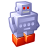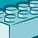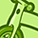# Integration, Extension & APIs

Announcements
Customer & Partners, DEC. 9, 11 AM ET: Qlik Product & Strategy Roadmap Session: Data Analytics REGISTER NOW
cancel
Showing results for
Did you mean:Not applicable

## R integration: time series forecast append

Hi everyone,

I am trying to append a forecasted time series to a line chart of closing values for a stock. Qlik Sense gives me  'erroe: client specified an invalid argument'. I don't understand what I'm getting wrong. Many thanks for your help.

R.ScriptEval('library(forecast);data<-ts(na.omit(q\$Variable1),frequency=1);fit<-auto.arima(data); res<-forecast(fit, level=0.95, h=q\$vForecastPeriods); n <- length(q\$Variable1); fore <- append(q\$Variable1[(q\$vForecastPeriods - 1):n],res\$mean,after= n - q\$vForecastPeriods); \$fore;', Sum(Close) as Variable1, vForecastPeriods)

Labels (1)

1 Solution

Accepted SolutionsCreator II

Hi Stan,

First, you're using a variable in your expression, you may want to try:

R.ScriptEval('library(forecast);'&

'data<-ts(na.omit(q\$Variable1),frequency=1);'&

'fit<-auto.arima(data);'&

'res<-forecast(fit, level=0.95, h=q\$vForecastPeriods); '&

'n <- length(q\$Variable1); '&

'fore <- append(q\$Variable1[(q\$vForecastPeriods - 1):n],res\$mean,after= n - q\$vForecastPeriods); '&

'\$fore;',

Sum(Close) as Variable1,

\$(vForecastPeriods) as  vForecastPeriods )

Second, to help you in troubleshooting check the logs for SSE2Rserve, this should give you an indication of what is wrong in the script.

Regards,

Bas

7 RepliesCreator II

Hi Stan,

Currently, the R extension expects the same number of rows as a result. So you will need to add empty rows for the forecast values into the data that you send to R.

Regards,

Bas.Not applicable
Author

Hi Bas,

Thanks for the advice. I thought that's exactly what I'm doing above: sending in TS to R with its original length and retrieving TS - ForecastPeriods + Forecasts. Further thoughts are appreciated.Creator II

Hi Stan,

First, you're using a variable in your expression, you may want to try:

R.ScriptEval('library(forecast);'&

'data<-ts(na.omit(q\$Variable1),frequency=1);'&

'fit<-auto.arima(data);'&

'res<-forecast(fit, level=0.95, h=q\$vForecastPeriods); '&

'n <- length(q\$Variable1); '&

'fore <- append(q\$Variable1[(q\$vForecastPeriods - 1):n],res\$mean,after= n - q\$vForecastPeriods); '&

'\$fore;',

Sum(Close) as Variable1,

\$(vForecastPeriods) as  vForecastPeriods )

Second, to help you in troubleshooting check the logs for SSE2Rserve, this should give you an indication of what is wrong in the script.

Regards,

BasNot applicable
Author

Hi Bas,

Many thanks. It was solved by

\$(vForecastPeriods) as  vForecastPeriods

However, I received error with \$fore. I just took that \$ out of your code and it worked like a charm.

Thanks again!

PS. Any ideas on how to integrate a fan chart are welcome.Partner

Hi Stan,

Can i have your sample for this? im facing difficulties of mapping back the appended value back with dimension.

Thanks.Contributor

Hi Bas,

I am a beginner in Qlik and R.

As you mentioned, that we need need to add empty rows for the forecast values that is sent to R, can you please let me know how can that be done ? And wont it hamper the other visualizations in Qlik ?